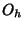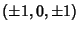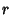## CuboctahedronAn Archimedean Solid (also called the Dymaxion or Heptaparallelohedron) whose Dual is the Rhombic Dodecahedron. It is one of the two convex Quasiregular Polyhedra and has Schläfli Symbol. It is also Uniform Polyhedronand has Wythoff Symbol. Its faces are. It has theOctahedral Group of symmetries.

The Vertices of a cuboctahedron with Edge length ofare,, and. The Inradius, Midradius, and Circumradius forareFaceted versions include the Cubohemioctahedron and Octahemioctahedron.The solid common to both the Cube and Octahedron (left figure) in a Cube-Octahedron Compound is a Cuboctahedron (right figure; Ball and Coxeter 1987).

See also Archimedean Solid, Cube, Cube-Octahedron Compound, Cubohemioctahedron, Octahedron, Octahemioctahedron, Quasiregular Polyhedron, Rhombic Dodecahedron, Rhombus

References

Ball, W. W. R. and Coxeter, H. S. M. Mathematical Recreations and Essays, 13th ed. New York: Dover, p. 137, 1987.

Ghyka, M. The Geometry of Art and Life. New York: Dover, p. 54, 1977.